# probability-120904030152-phpapp01.pdf

15 de Nov de 2022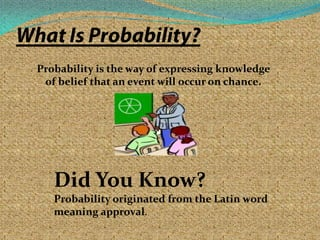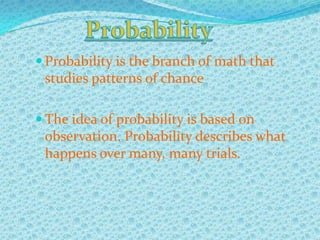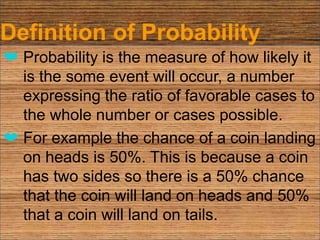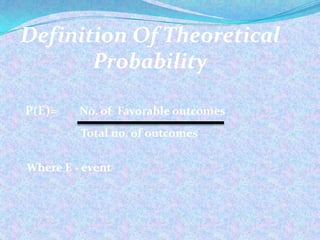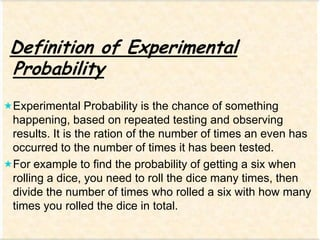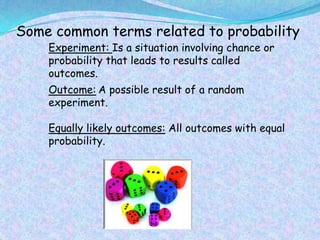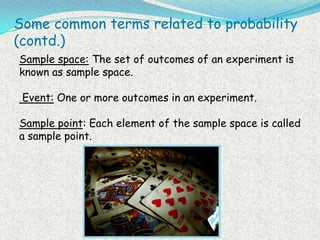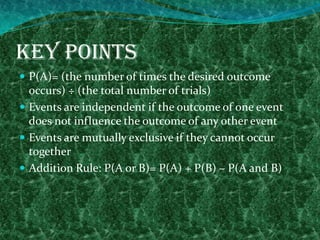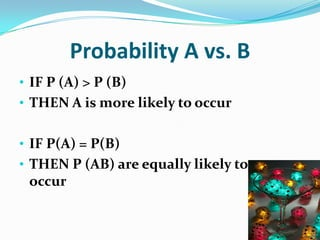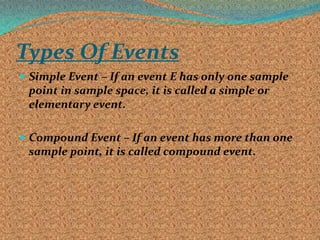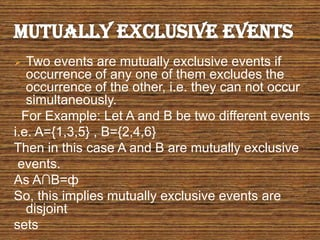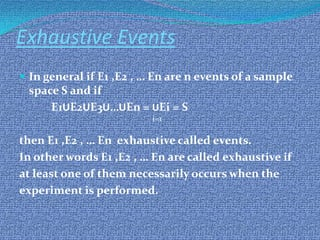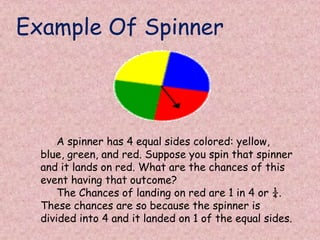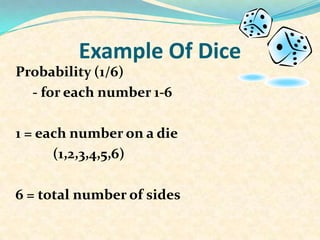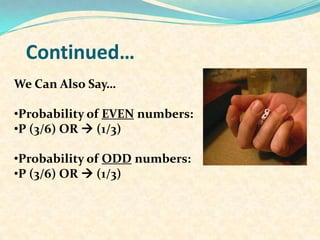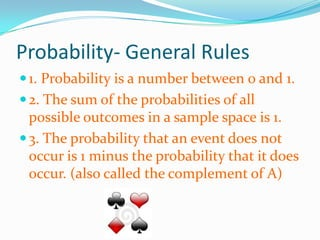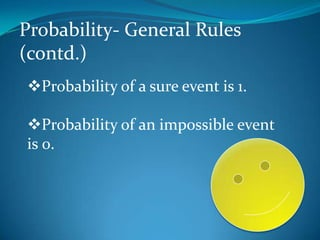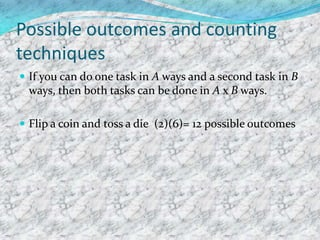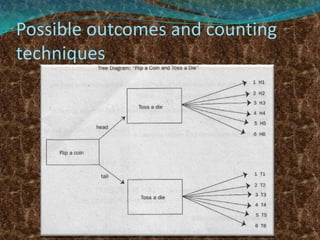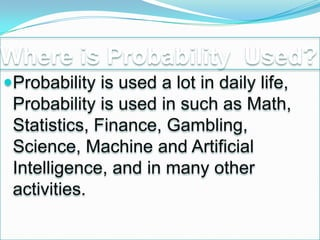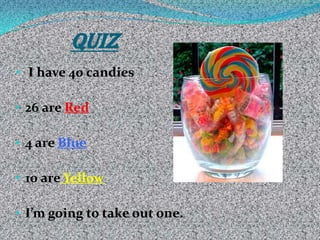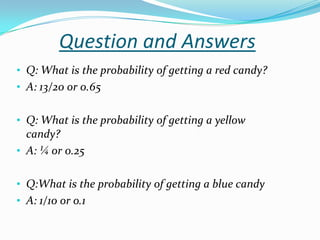1 de 25

### probability-120904030152-phpapp01.pdf

1. Probability is the way of expressing knowledge of belief that an event will occur on chance. Did You Know? Probability originated from the Latin word meaning approval.
2.  Probability is the branch of math that studies patterns of chance  The idea of probability is based on observation. Probability describes what happens over many, many trials.
3. Definition of Probability ❤ Probability is the measure of how likely it is the some event will occur, a number expressing the ratio of favorable cases to the whole number or cases possible. ❤ For example the chance of a coin landing on heads is 50%. This is because a coin has two sides so there is a 50% chance that the coin will land on heads and 50% that a coin will land on tails.
4. P(E)= No. of Favorable outcomes Total no. of outcomes Where E - event Definition Of Theoretical Probability
5. Definition of Experimental Probability Experimental Probability is the chance of something happening, based on repeated testing and observing results. It is the ration of the number of times an even has occurred to the number of times it has been tested. For example to find the probability of getting a six when rolling a dice, you need to roll the dice many times, then divide the number of times who rolled a six with how many times you rolled the dice in total.
6. When question arises related to chance of something happening , you are dealing with probability.
7. Some common terms related to probability Experiment: Is a situation involving chance or probability that leads to results called outcomes. Outcome: A possible result of a random experiment. Equally likely outcomes: All outcomes with equal probability.
8. Some common terms related to probability (contd.) Sample space: The set of outcomes of an experiment is known as sample space. Event: One or more outcomes in an experiment. Sample point: Each element of the sample space is called a sample point.
9. Key Points  P(A)= (the number of times the desired outcome occurs) ÷ (the total number of trials)  Events are independent if the outcome of one event does not influence the outcome of any other event  Events are mutually exclusive if they cannot occur together  Addition Rule: P(A or B)= P(A) + P(B) – P(A and B)
10. • IF P (A) > P (B) • THEN A is more likely to occur • IF P(A) = P(B) • THEN P (AB) are equally likely to occur Probability A vs. B
11. Types Of Events  Simple Event – If an event E has only one sample point in sample space, it is called a simple or elementary event.  Compound Event – If an event has more than one sample point, it is called compound event.
12.  Two events are mutually exclusive events if occurrence of any one of them excludes the occurrence of the other, i.e. they can not occur simultaneously. For Example: Let A and B be two different events i.e. A={1,3,5} , B={2,4,6} Then in this case A and B are mutually exclusive events. As A∩B=ф So, this implies mutually exclusive events are disjoint sets
13. Exhaustive Events  In general if E1 ,E2 , … En are n events of a sample space S and if E1UE2UE3U…UEn = UEi = S then E1 ,E2 , … En exhaustive called events. In other words E1 ,E2 , … En are called exhaustive if at least one of them necessarily occurs when the experiment is performed. i=1
14. Example Of Spinner A spinner has 4 equal sides colored: yellow, blue, green, and red. Suppose you spin that spinner and it lands on red. What are the chances of this event having that outcome? The Chances of landing on red are 1 in 4 or ¼. These chances are so because the spinner is divided into 4 and it landed on 1 of the equal sides.
15. Example Of Dice Probability (1/6) - for each number 1-6 1 = each number on a die (1,2,3,4,5,6) 6 = total number of sides
16. Continued… We Can Also Say… •Probability of EVEN numbers: •P (3/6) OR  (1/3) •Probability of ODD numbers: •P (3/6) OR  (1/3)
17. Probability- General Rules 1. Probability is a number between 0 and 1. 2. The sum of the probabilities of all possible outcomes in a sample space is 1. 3. The probability that an event does not occur is 1 minus the probability that it does occur. (also called the complement of A)
18. Probability- General Rules (contd.) Probability of a sure event is 1. Probability of an impossible event is 0.
19. Possible outcomes and counting techniques  If you can do one task in A ways and a second task in B ways, then both tasks can be done in A x B ways.  Flip a coin and toss a die (2)(6)= 12 possible outcomes
20. Possible outcomes and counting techniques
21. Where is Probability Used? Probability is used a lot in daily life, Probability is used in such as Math, Statistics, Finance, Gambling, Science, Machine and Artificial Intelligence, and in many other activities.
22. • I have 40 candies • 26 are Red • 4 are Blue • 10 are Yellow • I’m going to take out one. QUIZ
23. • Q: What is the probability of getting a red candy? • A: 13/20 or 0.65 • Q: What is the probability of getting a yellow candy? • A: ¼ or 0.25 • Q:What is the probability of getting a blue candy • A: 1/10 or o.1 Question and Answers